# CREST International IQ Challenge Class 9 Sample Papers

## Syllabus:

The IQ Challenge will evaluate students on the following skills:

• Analytical reasoning
• Calculation skills
• Creative thinking
• Information processing
• Lateral thinking
• Observation skills

The questions will be a mix of Basic, Intermediate and Advanced Level. It is advisable that students should attempt questions only if they know the answer. For every incorrect answer, there's a penalty of 1/3rd of the total marks allotted to that question.

 Q.1 Study the number series given below and answer the following question:5 1 1 5 1 4 7 5 1 2 8 4 1 4 7 8 6 8 6 9 0 3 0 1 4 7 9 5 2 How many such numbers are present in the above arrangement, each of which is immediately preceded by its factor and succeeded by an even number?
 Q.2 If the given figure is folded then which of the following cube is formed?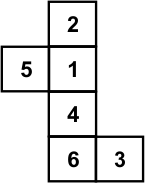Q.3 Find the missing number.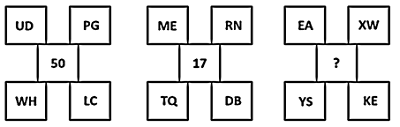Q.4 Read the following diagram carefully and answer the question:Who among the following is only a graduate?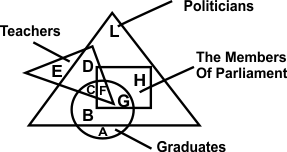Q.5 If PUMA = 8 and REEBOK = 12 then DECATHLON =?
 Q.6 Find the 3-D object whose different views are given below.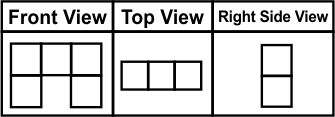Q.7 Choose the odd one out.
 Q.8 There are 5 people A, B, C, D and E who live in a same building. The building has 5 floors (where the lower most floor is numbered 1 and the floor above it is numbered 2 and so on). No two persons live on the same floor. B, C and D do not live in the lowermost floor. A lives on the 4th floor. C lives on the topmost floor. D does not live in the floor no.2.Who lives in floor number 3?
 Q.9 Find the missing number.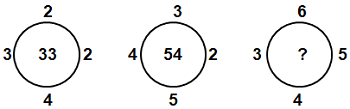Q.10 Which two signs and two numbers should be interchanged in the following equation to make it correct?(11 + 5 – 120 ÷ 4) × 20 = 40

Sample PDF of CREST International IQ Challenge for Class 9: